Orthogonal

Riemannian Quantum Mechanics

Orthogonal

The Structure of Solids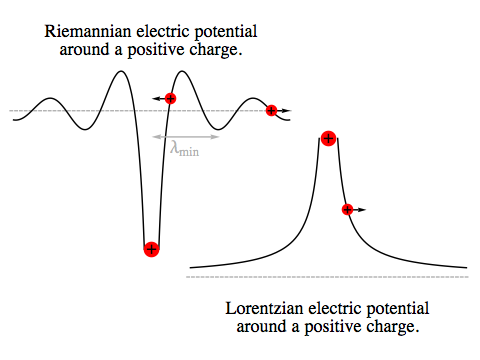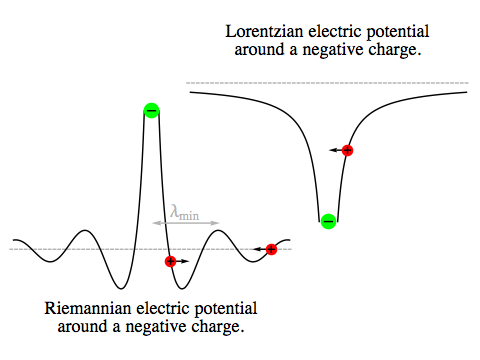In our universe, the electrostatic Coulomb force follows a simple inverse-square law. Like charges repel and opposite charges attract, so a positive charge in the vicinity of another positive charge will experience an “energy landscape” resembling a steep, smooth-sided mountain with a slope that gradually levels out with distance. Around a negative charge there’s an energy pit, the first landscape turned upside-down.

In the Riemannian universe, the equivalent force gives rise to a situation where, close up, like charges attract and opposite charges repel. But this is only true out to a certain distance, because the energy landscape around a charged particle has regularly spaced peaks and troughs superimposed on the inverse-square drop in the force.

The plots on the right show the energy as a function of distance. In the three dimensions of space around the central particle, the energy peaks and troughs will form concentric shells, creating the possibility for particles to become bound together by ending up in each other’s energy troughs.

But there’s a complication. If we analyse the situation with classical physics — that is, without taking account of quantum-mechanical effects — a particle in an energy valley is not going to be motionless, since it will usually possess enough energy to move back and forth to at least some degree. But in doing so, it will emit electromagnetic radiation. In our universe that would rob it of kinetic energy and damp down its motion, but in the Riemannian universe kinetic energy and the energy in electromagnetic radiation have the opposite sense, so a particle oscillating in an energy valley would be expected to move faster as it radiates. If it keeps gaining kinetic energy this way, it will eventually escape the valley completely.

One way a particle could remain bound would be if the natural frequency of its oscillations was larger than the maximum frequency of electromagnetic radition, νmax. A charged particle oscillating faster than νmax won’t produce radiation.

However, in order to produce oscillations with a single, pure frequency, an energy valley must have a perfect shape, where the potential energy as a function of distance from the centre is a flawless parabola. This is a very stringent requirement, and the Coulomb force certainly can’t meet it. For an “imperfect” energy valley, even if the main frequency of oscillation is higher than νmax there will also be lower-frequency components to the particle’s motion.

How, then, can any kind of matter be stable in the Riemannian universe? The answer lies in quantum mechanics. We’ll address the details shortly, but in order to do so we’ll need to formulate a simple, concrete example with which to demonstrate the effect.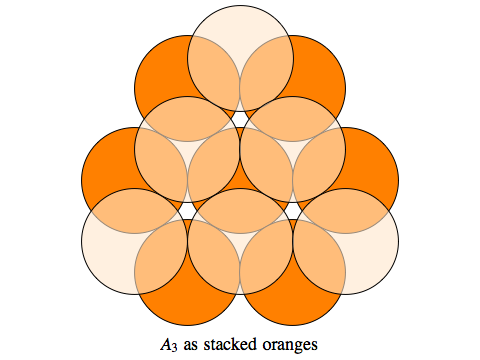Rather than looking at the Riemannian equivalent of a hydrogen atom, with just two particles bound by an electrostatic force, we’ll start at the other extreme and consider the energy landscape that one particle experiences when it is surrounded by a vast number of neighbours arranged in a regular array.

The particular arrangement we’ll consider is known by various names, including the “face-centred cubic system” to crystallographers, and the “A3 lattice” to mathematicians. If you take a grid of cubes and place a particle at every vertex of each cube and in the centre of every face, that gives an A3 lattice. Mathematically, if we take all points (x, y, z) where x+y+z is an even integer, that will produce a version of the lattice where the side of each cube has length 2, and the distance between a particle and its nearest neighbour is √2. Of course we can scale the whole lattice any way we please by multiplying everything by some factor s.

The blue planes in the diagram on the upper left illustrate another way of “slicing up” the same lattice. These planes are divided into equilateral triangles, with particles at their vertices. This arrangement is the natural way spherical objects such as oranges or billiard balls can be stacked in a plane, and the successive planes fit together just like successive layers of oranges fitting into the depressions in the layer below.

Each particle in the A3 lattice has twelve nearest neighbours: six in the same “layer of oranges”, three from the layer above and three from the layer below. Since the nearest neighbours lie at a distance of √2, their squared distance is 2. In our original scheme, every lattice point has coordinates (x, y, z) where x+y+z is an even integer, and since:

(x + y + z)2 – (x2 + y2 + z2) = 2 (xy + yz + xz)

the squared distance of (x, y, z) from (0, 0, 0) will also be an even integer. It follows that (0, 0, 0) has neighbours at distances of:

√2, √4, √6, √8, √10 ...

Every point in the lattice will have neighbours in the same configuration. The number of particles at successively larger distances is the number of ways of writing 2, 4, 6, 8, 10 ... as a sum of three squared integers (with zero and negative numbers allowed, and with different ordering of the terms counted separately). The first few counts are:

12, 6, 24, 12, 24, 8, 48, 6, 36, 24 ...

but there is no simple formula for this sequence.

Now, suppose we have a cluster of positively charged particles arranged in an A3 lattice. If the cluster had just 4 particles they could all be equidistant from each other, and we could choose the overall scale so that they were all in the dead centre of each others’ nearest energy trough. But for a large cluster, the particle-to-particle distances will include all the various neighbours’ distances we listed above, the square roots of all the even integers. Obviously these are not all integer multiples of any value, so it’s impossible to arrange for them all, individually, to lie at the bottom of an energy trough. So the overall lattice spacing will be a compromise which minimises the energy, balancing the advantages of having the nearest-neighbour distance close to the trough-to-trough distance λmin of the Coulomb potential against the effect that has on the greater number of neighbours at other distances.

It turns out that, for very large clusters, the potential energy of the cluster is minimised when:

s = (√3)/2 λmin ≈ 0.866 λmin

and the nearest-neighbour distance is:

d = (√2) s = √(3/2) λmin ≈ 1.2247 λmin

Since this is almost exactly one quarter-wavelength above λmin, the contribution to the energy from the nearest neighbours will be very small! Nevertheless, a sum that includes far more distant neighbours turns out to give the deepest energy well for this value of s.

It will be useful at this point to introduce the fine structure constant, α, a dimensionless quantity defined (in units where time and space are treated identically, as we’re doing throughout these notes) as:

α = e2 / (4 π ℏ ε0)

Here e is the charge of the particles from which the lattice is built, ε0 is the permittivity constant that determines the strength of the electrostatic force, and ℏ is Planck’s constant divided by 2 π. In our own universe, α ≈ 1/137, but of course there’s no reason why it should be the same in the Riemannian universe.

We will also define:

mphoton = ℏωm

The energy of each quantum of light of a given angular frequency is found by multiplying that angular frequency by ℏ. So ℏωm is the energy associated with light of the maximum possible angular frequency. But as we’ve seen when we looked at energy and momentum in the Riemannian universe, the maximum total energy of a particle is just its rest mass. So ℏωm is the rest mass of a Riemannian photon.

If we multiply these two constants together, the product αmphoton is equal to 2 π times the potential energy of two particles separated by a distance of λmin. This makes it a useful energy scale for any situation where the spacing of particles is tied to λmin.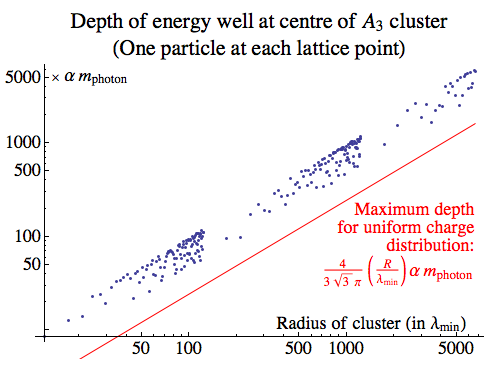The log-log plot on the right shows the depth of the energy well seen by a particle at the centre of a series of A3 clusters that are approximately spherical in shape. The vertical scale is in units of αmphoton, the horizontal scale in units of λmin.

The red line shown for comparison gives the deepest the well could be if the same charge were uniformly distributed throughout the same region. The exact depth, found by integrating the Riemannian Coulomb potential over a solid sphere, is oscillatory and cycles back and forth between this line and the opposite energy, where the well is a peak instead.

Not every cluster size has been sampled, so the oscillation of the energy as the cluster grows is tracked somewhat unevenly — but the clear trend is for the depth of the well to grow in proportion to the size of the cluster, on average doing about twice as well as the uniformly distributed charge:

Dcentre ≈ [8 / (3 √3 π)] (Rmin) αmphoton

This is not the kind of thing we’d expect in a crystalline solid in our own universe, where beyond a certain point the size of the crystal would make no difference to the conditions experienced by an electron deep in the interior! It might be tempting to think that this long-range influence must be due to the decision to build the lattice entirely out of positive charge, with no negative charges to cancel out their cumulative effect. But in fact, negative charges added to the lattice would make little difference in that respect: they would sit at their own lattice of energy minima — at points that looked like energy peaks to a positive charge — and the pattern of potential energy they created would actually deepen the energy wells for the positive charges.The depth of the energy well is important, but what about the shape? Any finite cluster of particles will have wells that aren’t perfectly rotationally symmetrical, and are different from site to site within the lattice, but it’s still useful to consider the idealisation of a rotationally symmetrical well, where the potential energy depends only on the distance from the centre. To approximate the shape of the well, we can make use of the potential energy curve inside a uniform spherical shell of charge; the total potential will be the sum of many such terms, but each will be a constant times:

Us(r) = –sin(ωm r) / (ωm r)

Inasmuch as we can approximate the lattice as a series of charged shells, the shape of the central well must be some fixed multiple of this Us(r).

Us(r) itself is, of course, not a parabola. The blue curve in the plot on the right shows Us(r), while the red curve is the parabola that shares the same second derivative at r=0. The “imperfection” in the shape of the well, even when we idealise it as rotationally symmetrical, means that a classical particle moving back and forth in this well will not have a single, pure vibrational frequency. So even if we ensure that the main frequency of its oscillations is greater than νmax, in classical physics that is not enough to prevent it radiating through lower-frequency components of its motion.

Imperfect Harmonic Oscillators

When a particle moves in an energy well where the potential energy (relative to the bottom of the well) is proportional to the square of the distance from the centre of the well, the force pulling the particle towards the centre will simply be proportional to the distance. A system like this is known as a harmonic oscillator. A weight vibrating on the end of a spring, or a pendulum swinging back and forth under gravity, are two examples of this — though as with most real physical systems, the energy well is only approximately parabolic, and the description works best when the oscillations are small.

Suppose we have a perfectly parabolic energy well, radially symmetric in three dimensions, in which a particle is moving without friction, radiation, or any other complications. If the constant of proportionality between force and distance is k, we have for the potential energy U and the force F:

U = (1/2) k r2
F = –∂r U er = –k r er

Let’s resolve this radial force into components pointing along the x, y and z axes. If the particle has coordinates (x, y, z), then the unit vector in the radial direction is:

er = (x/r, y/r, z/r)

which means the individual components of the force are simply:

(Fx, Fy, Fz) = (–k x, –k y, –k z)

Using force equals mass times acceleration to find the second rate of change with time of each coordinate, we have:

(∂t2 x(t), ∂t2 y(t), ∂t2 z(t)) = (–(k/m) x(t), –(k/m) y(t), –(k/m) z(t))

These three equations are solved by:

(x(t), y(t), z(t)) = (Ax cos(ω t + φx), Ay cos(ω t + φy), Az cos(ω t + φz))
where ω = √(k/m)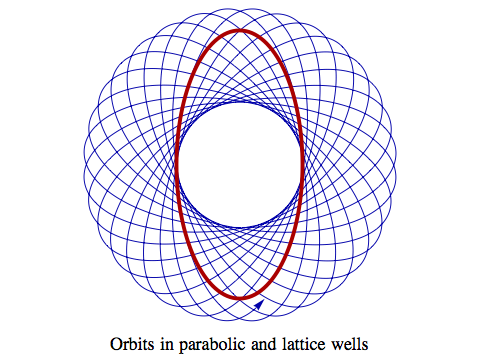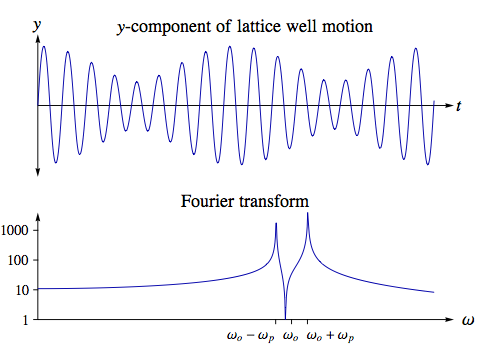The particle will oscillate back and forth along each coordinate direction with the same frequency ω in each case. The amplitudes of the oscillations, Ax, Ay and Az, will generally be different, as will the phases φx, φy and φz. But despite any differences in those parameters, because the frequency is the same, after a time 2 π / ω the particle will always end up back at exactly the same point. It executes a closed orbit, which in the general case will be an ellipse whose centre is the centre of the well, such as the red curve in the diagram on the right.

What happens if the energy well is not exactly parabolic? The force will no longer be exactly proportional to r, and the individual components of the force will no longer depend only on the corresponding coordinates. Everything becomes more complicated, and the only orbits that will be closed are circular ones, with no radial motion at all. The blue curve in the diagram on the top right shows a portion of an orbit in an energy well of the form Us(r), the function we’re using for the potential energy of a particle in a lattice.

The diagram on the lower right shows the y-component of the particle’s motion over time, along with a Fourier transform, which describes the frequencies from which the motion is composed. The fact that the orbit precesses — that is, the point where it is furthest from the centre of the well rotates over time, with an angular frequency we’ll call ωp — splits the strongest peak in the frequency spectrum from a single value at ωo, the orbital frequency (which is all there would be for a parabolic well) into two separate peaks, at ωo±ωp.

Now, these peaks are very high and narrow (note that the strength of these components is plotted on a logarithmic scale!), so if ωo–ωp > ωm, very little of the particle’s motion would be at frequencies that could radiate. But “very little” isn’t zero, and over time the particle would still gain energy and eventually be driven out of the well.

Quantum Effects in Solids

So far, our treatment has been entirely classical, but to really understand this situation we need to employ quantum mechanics. The classical physics of a moderately slow-moving particle in the Riemannian universe is described by Newtonian physics, just as it is in our own universe, and since non-relativistic quantum mechanics is the quantum version of Newtonian mechanics, we can make use directly of many of its results. In particular, for a parabolic energy well we have exactly the same behaviour for a quantum harmonic oscillator as in our universe.In quantum mechanics, the state of a particle is not described by a definite position and velocity, but by a wave function. This associates a complex number, known as the amplitude, to each point in space where the particle might be; the probability of finding the particle there is proportional to the squared magnitude of the amplitude.

Wave functions for which the particle possesses a definite energy have shapes that remain fixed over time. The plot on the right shows some examples. The complete wave functions vary over the three dimensions of space; what’s shown here is just their dependence on the distance from the centre of the well, r.

The numbers n and l are quantum numbers that classify the various possible wave functions for a three-dimensional quantum harmonic oscillator. The quantum number n is related to the total energy of the particle, with:

En = (n + 3/2) ℏω

Here n is a non-negative integer, ℏ is Planck’s constant divided by 2 π, and ω is the angular frequency at which an equivalent classical oscillator would oscillate. So the particle has a sequence of equally spaced energy levels. The lowest possible energy state, with n=0, doesn’t lie at the bottom of the well; rather, it is one-and-half steps of size ℏω up from there, after which all the other levels are one step apart.

The quantum number l describes the total amount of angular momentum the particle possesses. As can be seen from the wave-function plots, as l increases the particle is more likely to be found further from the centre of the well. What is remarkable about the harmonic oscillator, though, is that l has no effect whatsoever on the particle’s total energy. The quantum number l is restricted to lie between n and 0, and to be even if n is even and odd if n is odd. A third quantum number, usually called m, can take any integer value from –l to l, and specifies the amount of angular momentum along some chosen coordinate axis; m has no effect on either the energy or the radial wave function, but along with l determines the way the wave function varies at different angles from the axis.

All in all, there are (n+1)(n+2)/2 distinct quantum states that a particle can occupy in a three-dimensional harmonic oscillator, all with identical energy En.Now, when the energy well is not exactly parabolic, there are small changes in the shapes of all the wave functions. The most important consequence of this is that l, the angular-momentum quantum number, now does affect the total energy of the particle. So the levels that in the parabolic case consisted of a single value now split into a number of closely spaced levels.

The diagram on the right shows the energy levels for a selection of particle masses and well depths. The levels drawn in red in each case are those for a perfectly parabolic energy well, while the levels in blue are for a well of the same depth that takes the form Us(r), the approximate shape we expect for the potential energy of a particle in a lattice.

In quantum mechanics, a charged particle no longer emits radiation in the continuous manner that classical physics describes. Rather, if it is in a particular state with energy EA, and another state is available to it, with energy EB, it can emit a photon whose energy equals the difference between the two, and jump from state A to state B. This is how the electrons in an atom emit light, in our universe. But in Riemannian physics there are two differences. Firstly, if EA and EB are described using the convention that kinetic energy is positive, the photon energy has the opposite sense. So the transition will only take place when EB > EA, with the charged particle jumping to a higher energy level as it emits the photon.

The other difference is that, in Riemannian physics, we have the maximum possible photon energy, mphoton, to consider. If EBEA > mphoton, then no single photon can possess enough energy to enable the transition to take place. In principle the particle could emit two photons, or three, or four, but the probability of a transition becomes smaller and smaller the greater the number of photons required.

The upshot is, if a particle in an energy well is in a quantum state where the next-highest state is several multiples of the maximum photon energy above it, its condition will be stable. Very few situations in quantum mechanics are stable for all eternity, but the greater the energy gap, the more stable the status quo will be. For example, a particle in the lowest energy state in the first well in the diagram will have a gap of 5.2 mphoton above it, so it would require the simultaneous emission of six photons to jump to a higher state.

Crucially, the change from a parabolic energy well to the imperfect kind of shape we expect in a lattice need not undermine this stability. There is some shifting and splitting of the energy levels, but for many wells there are still levels with a gap above them of several multiples of the maximum photon energy. It doesn’t matter if there is a much smaller gap below one of these levels, which would allow a particle to absorb a photon from the environment and move down into a lower level, then re-emit the photon and jump back up to where it started. Such transitions simply let the solid interact with light, without having any adverse effect on its stability. (In fact there are some subtle issues determining which transitions can take place with a single photon even when the energy gap is less than mphoton.)

Exactly which solids will be quantum-mechanically stable will depend on a host of parameters, including the mass of the particles making up the lattice and the depth of the energy wells in the lattice. The depth of the wells will depend on the size of the lattice, the strength of the Riemannian electrostatic force, and the charge on each particle. Our earlier calculations showed that:

Dcentre ≈ [8 / (3 √3 π)] (Rmin) αmphoton

where R is the radius of the cluster, and Dcentre is the depth of a well at the very centre of the cluster. We’ll modify this formula to allow for two slight complications. Firstly, we want to ensure that even wells at the edge of the cluster are stable, which we’ll do by initially halving this estimate. Secondly, we want to allow for the possibility that there are several particles in each energy well. So we’ll multiply our estimate by W, the number of particles in each well, since each particle will contribute more or less equally to the field throughout the lattice.

D ≈ [4 W / (3 √3 π)] (Rmin) αmphoton

Of course as in our own universe, a realistic solid might consist of many small crystals, rather than maintaining a perfect lattice structure from end to end, in which case R would be the radius of one such crystal, not the whole solid mass.

Suppose the depth of a typical energy well in the lattice is D. The best parabolic approximation to the potential is then:

Up(r) = D [(1/6) ωm2 r2 – 1]

As well as sharing the depth D, this function shares the same second derivative at r = 0 as the lattice potential Us(r). The force on a classical particle in this well is given by:

F = –∂r Up(r) er = –(D/3) ωm2 r er

making the force constant k equal to (D/3) ωm2, and the natural frequency of oscillation for a particle of mass m in the well equal to:

ω = √(k / m) = ωm √[D/(3m)]

This means that the ratio between the size of the energy steps in the well, ℏω, and the maximum photon energy mphoton = ℏωm, is given by:

(ℏω)/mphoton = ω/ωm = √[D/(3m)]

So, ignoring the shifts and splitting of levels, the lattice’s stability will depend on the value of √[D/(3m)]. If √[D/(3m)] ≤ 1, the lattice will not be stable, whereas the greater this quantity, the more stable the lattice will be. The diagram illustrates this effect, with the energy levels for different values of n coming closer together when either the particle mass m is increased or D is decreased.

If we want to guarantee that:

(ℏω)/mphoton = √[D/(3m)] > S

for some “safety factor” S, the particle-mass-to-photon-mass ratio must be less than a critical value, shown in (1a). If that ratio is taken to be fixed, we can achieve the same level of stability if the size of the lattice is greater than the threshold shown in (1b).

 Lattice stability conditions m/mphoton < [4 W α / (9 √3 π S2)] (R/λmin) (1a) R/λmin > [9 √3 π S2 / (4 W α)] (m/mphoton) (1b)

A smaller particle mass is good for stability, because this increases the gap between energy levels, so more photons are needed to bridge that gap. But it’s possible for the mass to be too low. A perfect harmonic oscillator has an energy well that stretches on forever, with the walls simply climbing higher and higher, but the wells in the lattice have a finite width and depth. If the energy gap is too great compared to the depth of the well, the particles in the well that occupy the highest energy levels risk jumping not to a higher bound state but right out of the well. In fact if the levels are spaced too far apart we might not even have the W states we need inside the well.

Assuming the particles making up the lattice are fermions, so that like electrons in our own universe they can’t share the same quantum state, the W particles will fill several energy levels. There are (n+1)(n+2)/2 distinct states in a quantum harmonic oscillator for each value of the quantum number n, but if we also count states of different spin there are twice that many, so the total number of states up to and including any given n is:

Σi=0n (i+1)(i+2) = (n+1)(n+2)(n+3)/3

Solving this precisely for the highest energy level occupied by a total of W particles is very messy, but we can make a rough approximation and say:

n3√(3 W)

The requirement that the nth energy level fits in the well puts a lower limit on the mass ratio, which is given below in (1c), with the equivalent limit on R as (1d).

Now, as the lattice grows larger the floor on the mass, (1c), gets lower and the ceiling on the mass, (1a), becomes higher, so whatever the values of α and S a sufficiently large lattice will be stable. Conversely, though, for a small enough lattice we’ll reach a position where the ceiling meets the floor and it’s impossible to satisfy both conditions at once. Condition (1e) is the requirement that the lattice is large enough that this isn’t the case.

 Lattice stability conditions, continued m/mphoton > [37/6 π / (4 α 3√W)] (λmin/R) (1c) R/λmin > [37/6 π / (4 α 3√W)] (mphoton/m) (1d) R/λmin > 311/6 π S / (4 W2/3 α) (1e)As an example, if α=1/137 (the value of the fine structure constant in our own universe), and if S=4 (so we’re ensuring that all particles would need to emit at least four photons simultaneously in order to jump out of their ground states), condition (1e) requires:

Rmin > 3225 / W2/3

The plot on the right gives a rough picture of the range of possibilities for stable lattices. We’ve ignored the effects of level-splitting, which can be destabilising for the highest energy levels, and we’ve also neglected to take account of the interaction between particles in the same well, which could improve stability by lowering some of the energy levels. Nevertheless, the general trend that’s apparent here should hold: for a given particle mass, adding more particles to each well allows smaller lattices to be stable.

Riemannian Atoms?

Having considered a lattice containing millions of particles, let’s now analyse a system of just two identical particles. In principle, there are two distinct ways in which they might be bound together. They might be separated by a distance of roughly λmin, putting each one in the other’s first “energy trough”. Alternatively, they might be closer than λmin/4, which would put them in each other’s “energy pit”, the bottomless well of electrostatic attraction that occurs before the electrostatic force starts switching back and forth between attraction and repulsion.

But we can see from our calculations on lattices that a pair of particles is unlikely to be stable if they’re sitting in each other’s energy troughs. Although the details of the situation are different from those in a lattice, the same general factors influence the depth of the energy well and the spacing of the energy levels. Condition (1e) for W=1 and Rmin becomes:

α > (311/6 π / 4) S ≈ 5.89 S

Even assuming that we’re free to pick the values of all the fundamental constants in the Riemannian universe, if we choose a large value for α in order to make this true, it will just increase the rate at which particles jump from one energy level to another, requiring us to choose a larger value for S in order to ensure stability.

If the two particles are much closer, though, we can forget about the corrugations in the Coulomb force entirely and treat the electrostatic attraction as an inverse-square force. The energy levels of a quantum-mechanical system like this are extremely well known, because apart from a few changes to some constants they’re just the energy levels of a hydrogen atom. Of course a hydrogen atom consists of a negatively charged electron bound to a much heavier, positively-charged proton, but the mathematics of the wave functions, determined by the inverse-square attraction, is essentially the same in both cases.

If we adapt the quantum mechanics of the hydrogen atom, we find that the energy levels of the simplest, two-particle Riemannian atom are given by:

E1 = –m α2 / 4
En = E1 / n2

Here m is the mass of each particle, α is the fine structure constant, and the quantum number n is an integer greater than or equal to 1. In principle for the hydrogen atom there is no upper limit on the value for n, but for our Riemannian atom if n becomes too great the wave function will stretch beyond the energy pit and the approximation we’re using of an inverse-square force will cease to apply. Indeed, we need this to be a reasonable assumption at least up to n=2. The distance scale for the wave function can be found by adapting what’s known as the Bohr radius for the hydrogen atom; the appropriate value for our scenario is:

a0 = 2 ℏ / (m α)

For the n=2 wave function to be sufficiently localised, we need:

a0 < λmin / 64

The factor of 1/64 here is somewhat arbitrary, but it puts the probability of the particles being more than λmin / 4 apart (the point where the potential first crosses zero) at less than 0.1%. This corresponds to a condition on the ratio of the particle mass to the photon mass:

 Two-particle atom, stability conditions m/mphoton > 64 / (π α) (2a)

Now, what is the condition for this atom to be stable, in its n = 1 state? The difference between the first and second energy levels is:

ΔE = E2E1 = (–3/4) E1 = 3 m α2 / 16

The crucial quantity is how many photon masses this energy difference comprises:

ΔE / mphoton = [3 α2 / 16] [m/mphoton]

For the atom to be safe from spontaneous photon emission, we’ll want this ratio to exceed some safety factor S, and so:

 Two-particle atom, stability conditions continued m/mphoton > 16 S / (3 α2) (2b)

For S=4 and α=1/137, this means m/mphoton > 400,000! So a two-particle atom requires a far more massive particle than the kind that would make a reasonably-sized lattice stable.

If we have Z particles in the atom instead of just two, the energy levels will be multiplied by Z2. However, assuming these particles are fermions, the Z particles will fill several energy levels. There are 2n2 distinct quantum states with quantum number n, so the top n value is given by:

Z = 2 (1 + 4 + 9 + ... + n2) = n (n+1) (2n+1) / 3 ≈ 2n3 / 3
n3√(3Z/2)

(Note that these calculations are rough approximations for large Z only, and they can’t be applied to our two-particle atom.) Rather than needing a safe gap between n=1 and n=2, we’ll need one between n and n+1:

ΔE = Z2 (En+1En)
= Z2 (– E1) [1/n2 – 1/(n+1)2]
= Z2 (m α2 / 4) [2n+1] / [(n+1)2 n2]
≈ 2 Z2 (m α2 / 4) / n3
Z m α2 / 3

Requiring a safety factor of S for this energy gap means:

 Z-particle atom, stability condition (approximation for large Z) m/mphoton > 3 S / (Z α2) (3)

For S=4 and α=1/137, even an atom with Z=1000 would require m/mphoton > 225.

Macromolecules

In between the case of a solid, crystalline lattice and a Riemannian atom, there should be a range of structures that can be built from particles of any given mass. The most obvious criterion for stability is that the energy levels for the individual particles include states that are isolated above by a sufficient gap, and we’ve seen that deepening the energy wells by various means can achieve this, but to do so might require incorporating hundreds or thousands of individual particles into the structure.

For a system of particles to be stable, it will also be necessary that the energy levels for the system as a whole have the same kind of gaps, so that collective vibrations, flexing and rotating of the “molecule” are also protected from runaway light generation. Rotational states have energies that are proportional to the square of the associated quantum number, and since the squares of the integers grow increasingly far apart, the rotational energy spectrum will eventually have very large gaps above each state. Vibrations and flexing motions are trickier, and on top of the need for suitable gaps in the energy spectrum for each individual degree of freedom of the molecule, it will be necessary that the structure has enough symmetry that the full list of energy levels doesn’t end up as a random-looking thicket of closely spaced lines: a set of a thousand identical oscillators, all with exactly the same energy gap of ΔE, will have an energy spectrum whose smallest gap is ΔE, but a set of a thousand oscillators with energy gaps that vary between them could gain a smaller amount of energy than the quantum for any individual oscillator, by simultaneously having one oscillator jump to a higher level while another falls to a lower level.

Characterising exactly which molecules will be stable against all of these threats would be a major feat in computational quantum chemistry, so all we can do is paint a broad picture. Large, polymer-like, perhaps even sheet-like molecules would stand the best chance, and the result could be that the most common states of matter apart from dilute gases all have a relatively high degree of long-range order. So rather than the seething liquids of small molecules interacting at random that we’re familiar with, the Riemannian universe might have much of its chemistry taking place in something more like liquid crystals. The regular arrangement of energy wells that can be seen within a lattice actually extends some distance beyond the lattice itself, and the same could be true of many stable large molecules, so that their interactions would involve them ratcheting along side-by-side, slipping in and out of each other’s serrations in the energy landscape.

There is extra material on this topic for readers who don’t mind a slightly higher level of mathematics.Orthogonal / Riemannian Quantum Mechanics / created Wednesday, 6 April 2011